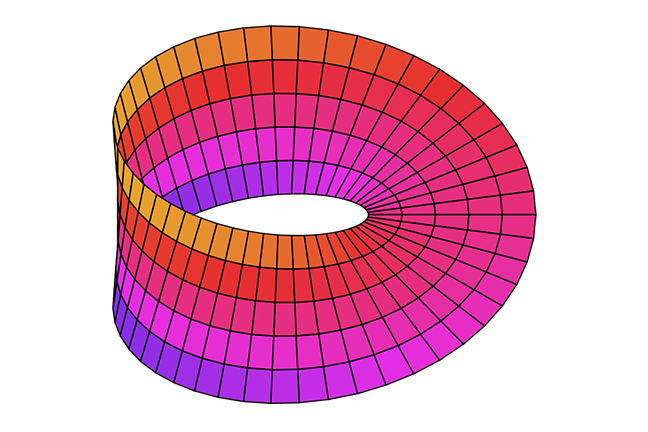## Want to keep learning?

This content is taken from the UNSW Sydney's online course, Maths for Humans: Inverse Relations and Power Laws. Join the course to learn more.
2.8

## UNSW SydneyA one-sided surface

# Translating hyperbolas rationally

What happens when we take the graph of $$\normalsize{y=\frac{1}{x}}$$ and start to move it around by scaling it and translating it? We obtain an interesting and important family of functions, called Mobius transformations. These are special kinds of rational functions.

In this step we

• see how algebra and transformations combine when moving around the hyperbola $$\normalsize{y=\frac{a}{x}}$$ to get a Mobius transformation

• introduce the idea of a rational function.

## Ferdinand Mobius and his transformations

Ferdinand Mobius (1790-1868) was a German geometer and astronomer who was a co-discoverer, along with J. Listing, of the Mobius band, a lovely one-sided surface.

Q1 (M): Here is a classic question. Make yourself a Mobius band, just by taping the ends of a strip of paper together, after having introduced one twist in it. What do you think will happen if you cut this surface down the middle? You might like to try to visualise it first. The trick is to figure out exactly what you get.

## Translating the standard hyperbola

Although Mobius transformations are defined as quotients of linear or constant polynomials, they also have an alternate description. It turns out that if we translate the curve $$\normalsize{y=\frac{a}{x}}$$, then we get exactly a Mobius transformation, and furthermore every Mobius transformation has this form. That means that the graphs of Mobius transformations are just translates of the graphs of standard inverse relations.

So graphs of Mobius transformations are geometrically just hyperbolas, and are therefore conics. We can see this algebraically as well since they have second degree equations once you clear denominators.

For an example, let’s start with $$\normalsize{y=\frac{1}{x}}$$, and translate it by $$\normalsize{3}$$ in the negative $$\normalsize{x}$$ direction this time. We get the function $$\normalsize{y=\frac{1}{x+3}}$$. Now suppose we translate this by $$\normalsize{2}$$ in the $$\normalsize{y}$$ direction, to get $$\normalsize{y=\frac{1}{x+3}+2}$$. By getting the right hand side under a common denominator, we end up with the Mobius transformation

$\Large{y=\frac{2x+7}{x+3}}.$

Here is what the graph looks like:Note that the asymptotes of the graph are now the vertical line $$\normalsize{x=-3}$$ and the horizontal line $$\normalsize{y=2}$$. This is not a surprise: when we translate by $$\normalsize{-3}$$ in the $$\normalsize{x}$$ direction, we should expect that the asymptotes also translate by $$\normalsize{-3}$$ in the $$\normalsize{x}$$ direction, and similarly when we translate in the $$\normalsize{y}$$ direction.

Q2 (E): What do you get when you start with $$\normalsize{y=\frac{4}{x}}$$, then translate by $$\normalsize{2}$$ in the $$\normalsize{x}$$ direction, and by $$\normalsize{-1}$$ in the $$\normalsize{y}$$ direction?Q3 (M): What happens when you start with $$\normalsize{y=\frac{1}{2x}}$$, translate by $$\normalsize{5/2}$$ in the $$\normalsize{x}$$ direction, and then translate by $$\normalsize{-3}$$ in the $$\normalsize{y}$$ direction?## Where are the asymptotes?

In the original example

$\Large{y=\frac{2x+7}{x+3}}$

the vertical asymptote can be identified as the value of $$\normalsize{x}$$ where the denominator has the value $$\normalsize{0}$$, that is the line $$\normalsize{x=-3}$$. As $$\normalsize{x}$$ approaches this value, $$\normalsize{y}$$ gets either very big in a positive sense or very big in a negative sense. When $$\normalsize{x=-3}$$ the function is undefined.

We can also determine the horizontal asymptote by asking what happens to $$\normalsize{y}$$ if $$\normalsize{x}$$ gets very big positive or very big negative.

For example, suppose that $$\normalsize{x=1,000,000}$$. Then $$\normalsize{y=\frac{2,000,007}{1,000,003}}$$. This is quite close to $$\normalsize{2}$$. You can check that the same thing happens when $$\normalsize{x=-1,000,000}$$: the $$\normalsize{y}$$ value is still very close to $$\normalsize{2}$$. So the line $$\normalsize{y=2}$$ is the horizontal asymptote for this function.

## Rational functions

Next to polynomial functions, the simplest broad family of functions in mathematics are the rational functions: these are quotients of polynomials. For example

$\Large{y=\frac{x^5-3x+1}{x^2(x-1)(x+2)}}$

has graphWe see that such a function has a much more complicated shape than a simple polynomial. In particular there are vertical asymptotes, corresponding to where the denominator is equal to zero, and in this case the behaviour as $$\normalsize{x}$$ gets large appears to approximate the function $$\normalsize{y=x}$$.

So going from studying polynomials to rational functions is a big step! Fortunately the Mobius transformations act as an intermediate family of functions which makes the transition a lot smoother!

Q4: (E) Which of the following are Mobius transformations?
a) $$\normalsize{\dfrac{2x+1}{x^2-3}}$$

b) $$\normalsize{\dfrac{3x-5}{7x+1}}$$

c) $$\normalsize{\dfrac{x-20}{x}}$$

d) $$\normalsize{\dfrac{2}{x+1} + 3x}$$

A1. It should be reasonably obvious that you get a single long strip, since the original Mobius band only had one edge, which we were not cutting. However a more delicate issue is: what kind of single long strip do we get – is it another Mobius band, just longer, or something else? Tell us in the comments.

A2. When we translate $$\normalsize{y=4/x}$$ by $$\normalsize{2}$$ in the $$\normalsize{x}$$ direction and by $$\normalsize{-1}$$ in the $$\normalsize{y}$$ direction, the equation is $$\normalsize{y+1=\frac{4}{x-2}}$$, which is

$\Large{y=\frac{-x+6}{x-2}}.$

A3. When we translate $$\normalsize{y=\frac{1}{2x}}$$ by $$\normalsize{5/2}$$ in the $$\normalsize{x}$$ direction, we get the equation

$\Large{y=\frac{1}{2(x-5/2)}}.$

Then translating by $$\normalsize{-3}$$ in the $$\normalsize{y}$$ direction, we get

$\Large{y=\frac{1}{2(x-5/2)}-3}$

which simplifies to

$\Large{y=\frac{-6x+16}{2x-5}}.$

A4. The functions b) $$\normalsize{\frac{3x-5}{7x+1}}$$ and c) $$\normalsize{\frac{x-20}{x}}$$ are Mobius transformations. But a) $$\normalsize{\frac{2x+1}{x^2-3}}$$ is a rational function, but not a Mobius transformation since the denominator has degree two, and similarly d) $$\normalsize{\frac{2}{x+1} + 3x}$$ is a rational function but not a Mobius transformation because it can be rewritten as

$\Large{\frac{2}{x+1} + 3x=\frac{3x^2+3x+2}{x+1}}.$